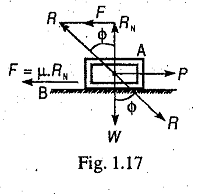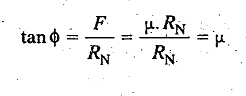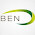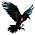### Limiting Angle of Friction

Limiting Angle of Friction is defined as the angle which the resultant reaction (R) makes with the normal reaction (RN).Consider a body A of weight W resting on a horizontal plane B as shown in Fig. 1.17. If a horizontal force P is applied to the body, no relative motion takes place until the applied force P is equal to the force of friction F, acting opposite to the direction of motion. The magnitude of this force of friction is
F = µ W = µ.RN, where RN is the normal reaction.

In the limiting case, when the body just begins to move, it is in equilibrium under the action of the following three forces :

1. Weight of the body (W),
2. Applied horizontal force (P), and
3. Reaction (R) between the body A and the plane B.

The reaction R must, therefore,be equal and opposite to the resultant of W and P and will be inclined at an angle (ϕ) to the normal reaction (RN). This angle ϕ is called the limiting angle of friction or simply angle of friction. From Fig. 1.17, we find that1.What is the physical significance of limiting of friction?

2.What is the physical significance of limiting of friction?

1.Hey ben johns. Im a novis at this stuff but limiting friction is significant as it is the max. possible friction btw 2 surfaces so im guessing calculations can be made using this max friction for safety certainty.

2.Idk much but i think Limiting fricion is the max possible friction so this value is impt for calculation for safety certainty

3.What is R...it is resultant btw Rñ&F or btw W&P??